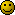# ecg signal

edited February 2008
hello everyone,

im doing a project about removing 50hz noise from ecg signal..now i have two codings in C++ for low pass filter and kaiser window..but i dont know how to implement this coding into matlab..i really dont have idea how to put all together in matlab and make it work..please help me..

loga

• edited January 2008
Can you use the filter design toolkit in Matlab?
• edited January 2008
im not very familiar with mathlab..im just going thorugh it..im kind of stucked in half way..
• edited January 2008
Well, Matlab syntax is very similar to C++. You can step through your C++ code and write it in Matlab.

An alternative would be to simply run the C++ code and then deal with the output data in Matlab.
• edited January 2008
is there any other way for me to eliminate the noise?like mathlab coding?
• edited January 2008
Yes, there are filter commands. Look through the matlab help or in your textbook• edited January 2008
why do you want to use matlab if you have c++ code? Or doesn't it do what you want?
• edited January 2008
loga wrote:
is there any other way for me to eliminate the noise?like mathlab coding?

I believe if you feed the signal through an operational amplifier you can eliminate the noise. I did have a circuit for it a few years back but where that is now???
A 741 differential amp with one input to the ECG signal, one open to the noise and the noise gets canceled out.
• edited January 2008
50hz noise might be from the mains power. it's likely that it would be tough to build a subtraction op-amp that would also scale the voltage to just the right amplitude.

if it is, it's simple to code up a notch filter in matlab.
• edited January 2008
the problem is i dont know how to make all this work in mathlab..i mean now i have a signal with noise..and then the coding given by my lecturer..im kind of stucked how to put all together in mathlab and make it works..
• edited January 2008
so, what do you want from us?
• edited January 2008
now i have problem with my coding..the code given by my lecturer is this..she asked us to understand this coding..we cant run it because of the header file..we dont have the function for the header file..that means we have to rebuild the code..but we really dont have idea how to do it..
``````// nonrec.cpp -- non-recursive filter design

//     NRLowPass()          build NR low-pass filter
//     NRHighPass()         build NR high-pass filter
//     NRBandPass()         build NR band-pass filter

#include "tools.h"
#include "ltisys.h"

// sinc() or sin(x)/x function
double sinc(double x)
{
if (x==0)
return cos(x);
else
return sin(x)/x;
}

// Create non-recursive low-pass filter by Fourier Transform method
LTISystem NRLowPass(
double freq,         // corner freq (fraction of sampling rate)
int ncoeff           // # coefficients
)                    // returns LTI system to specification
{
int    i;

// convert frequency
double omega = 2 * PI * freq;

// build half-sized window from sinc function
int nhalf = ncoeff/2;
Waveform hwv(nhalf,1.0);
for (i=1;i<=nhalf;i++)
hwv[i] = omega * sinc(i*omega)/PI;

// window with (half-)hamming window
for (i=1;i<=nhalf;i++)
hwv[i] *= 0.54 + 0.46 * cos(i*PI/nhalf);

// create new LTI System
LTISystem lpfilt(2*nhalf,0);

// copy impulse response into system
//   (indexed -nhalf .. 0 .. nhalf)
lpfilt.a[nhalf] = omega/PI;
for (i=1;i<=nhalf;i++) {
lpfilt.a[nhalf-i] = hwv[i];
lpfilt.a[nhalf+i] = hwv[i];
}

return lpfilt;
}

// create high-pass non-recursive filter from low-pass prototype
LTISystem NRHighPass(
double freq,         // corner freq (fraction of sampling rate)
int ncoeff           // # coefficients
)                    // returns LTI system
{
// get low-pass version
LTISystem hpfilt = NRLowPass(0.5-freq,ncoeff);

// now modulate with cos(n*PI) = +1,-1,+1,-1,...
int nhalf = hpfilt.a.count()/2;
for (int i=1;i<=nhalf;i+=2) {
hpfilt.a[nhalf-i] = -hpfilt.a[nhalf-i];
hpfilt.a[nhalf+i] = -hpfilt.a[nhalf+i];
}

return hpfilt;
}

// create band-pass non-recursive filter from low-pass prototype
LTISystem NRBandPass(
double lofreq,       // low corner freq (fraction of sampling rate)
double hifreq,       // high corner freq (fraction of sampling rate) }
int ncoeff           // # coefficients
)                    // returns LTI system
{
// get low-pass prototype
LTISystem bpfilt = NRLowPass((hifreq-lofreq)/2,ncoeff);

// now modulate with cos(n*centrefreq)
int nhalf = bpfilt.a.count()/2;
double cf = 2*PI*(lofreq+hifreq)/2;
bpfilt.a[nhalf] = 2 * bpfilt.a[nhalf];
for (int i=1;i<=nhalf;i++) {
bpfilt.a[nhalf-i] = 2 * bpfilt.a[nhalf-i] * cos(i*cf);
bpfilt.a[nhalf+i] = 2 * bpfilt.a[nhalf+i] * cos(i*cf);
}

return bpfilt;
}
``````
• edited January 2008
Do you understand what the code that she gave you is doing? That's the first step, i suppose. The first section is creating an FIR lowpass filter using the windowing method.
the second and third are creating FIR band/high pass filters from a low pass filter using modulation.

It sounds to me like you don't understand matlab at all, and what you want from me is to give you code that replicates this.
also, if you want to post code in the future, place it inside [php]
``````code goes here
``````
[/php] tags.
• edited February 2008
hello sir,

at first i really didnt understand matlab at all but im learning.i didnt ask you for the code which replicates the code given by lecturer..anyhow thanks for your reply..